telescopeѲptics.net          ▪▪▪▪                                             CONTENTS

# 8.2.1. Two-mirror telescope aberrations

The basic concept used for more detailed presentation of two-mirror aberrations is that given in "Astronomical Optics" from Daniel J. Schroeder. It requires only two parameters for computing system aberrations: one is secondary magnification m and the other, alternatively, either relative back focal distance η, or the height of marginal ray at the secondary (i.e. minimum relative secondary size) k (FIG. 122). The former is defined as the primary-to-final focus separation in units of the primary focal length, or η=B/ƒ1=(m+1)k-1, where B, ƒ1 and m are the back focal length in units of the primary mirror's focal length, primary's focal length and secondary's magnification, respectively, and the latter by k=1-s/ƒ1., with s being the primary-to-secondary separation (both ƒ1 and s numerically negative according to the sign convention).

Use of parameters in their relative ("dimensionless") form generally simplifies the relations, also making calculation quicker and more convenient by leaving out large numbers (focal length, radii, etc.).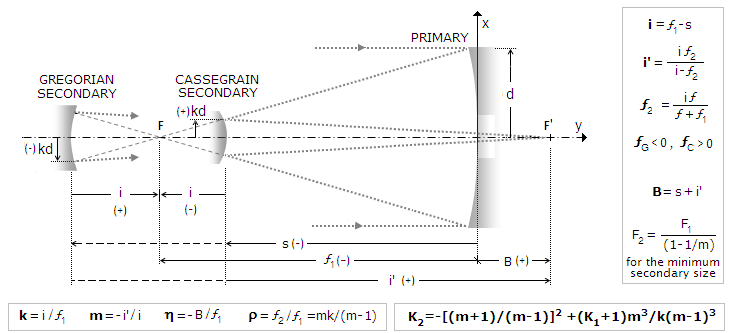FIGURE
122
:Two-mirror system in the right-hand Cartesian coordinate system. The main system parameters are the primary mirror focal length ƒ1, mirror separation s, system focal length ƒ (numerically negative for the Gregorian, positive for Cassegrain) and back focus distance B or η, when expressed in units of the primary's focal length. These three parameters determine relative height of marginal ray at the secondary k, in units of the aperture radius, secondary-to-prime-focus separation i, secondary focal length ƒ2 and magnification m, as well as secondary-to-final-focus separation i'. With the radii and separations known, mirror conics needed to correct aberrations can be determined; the relation bottom right determines primary (K1) and secondary conic (K2) needed to cancel spherical aberration. Sign of the linear parameters is determined by their direction: it is positive for left-to-right and upward from the axis, negative for the opposite directions. The negative sign in the relation for secondary magnification is due to magnification being defined as the ratio of image vs. object height. Since the object for the secondary is the image formed by the primary - reversed below the axis - secondary located inside the focus of the primary (Cassegrain arrangement), which forms reversed image below the axis, has positive magnification, while secondary located outside the focus (Gregorian), forming an erect image above the axis, has, by definition, negative magnification of the primary's image.

According to the sign convention, secondary magnification m is positive for the Cassegrain, and negative for the Gregorian, while the relative back focal distance η is positive for the final focus to the right from the primary mirror, and negative for the final focus to the left.

Following table details two-mirror system parameters determining its geometric and optical properties.

 PARAMETER RELATIONS Dimensionless w/actual system values ▪ secondary magnification m m=ρ/(ρ-k)= 1/(1-k/ρ) m=R2/(R2-R1+2s) ▪ height of marginal ray at the secondary in units of the aperture radius, k k=(1+η)/(m+1) k=1-(s/ƒ1)=1-(2s/R1) ▪ secondary to primary radius of curvature ratio, ρ=R2/R1 ρ=mk/(m-1) ρ=(ƒ1-s)m/(m-1)ƒ1 ▪ secondary radius, R2 R2=ρR1=mkR1/(m-1) R2=(R1-2s)m/(m-1) back focus distance (from the primary), η η=(m+1)k-1 η=[(R1-2s)R2/(R2-R1+2s)R1]-(2s/R1)

Note that k and ρ are also positive for the Cassegrain and negative for the Gregorian. With the Cassegrain, the secondary will form real image with ρ>k; for ρ=k, the secondary will produce collimated beams, and for ρ<k reflected beams will be diverging (i.e. magnification is numerically negative). Likewise, with the Gregorian, the condition for the secondary to form real image is |k|>|ρ|, with positive magnification values indicating secondary too weak to form a real image.

Two-mirror system parameters , with respect to their sign, are summarized in the following table.

 System R1, ƒ1 R2, ƒ2 ƒ s i i' B k ρ m η Cassegrain - - + - - + "+" for m>(1/k)-1  "-" for m<(1/k)-1 + + + "+" for m>(1/k)-1 "-" for m<(1/k)-1 Gregorian - + - - + + "+" for m<(1/k)-1  "-" for m>(1/k)-1 - - - "+" for m<(1/k)-1 "-" for m>(1/k)-1

TABLE 9: Numerical sign for main parameters of the Cassegrain and Gregorian two-mirror systems.

General aberration coefficients for the two-mirror telescope are obtained as a sum of aberration coefficients for the primary (with the stop at the surface) and secondary mirror (with the stop at the primary). For cancelled lower-order spherical aberration in a two-mirror system, for object at infinity, primary and secondary mirror conics - K1 and K2, respectively - have to relate as: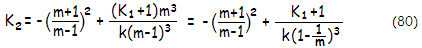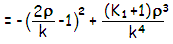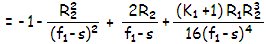This relation is derived by setting a sum of the spherical aberration coefficients for the primary (Eq. 9.2) and secondary mirror (Eq. 9), equal to zero. The sum itself, from Eq. 7, determines expression for the system P-V wavefront error of spherical aberration at the best focus for two-mirror systems in general as: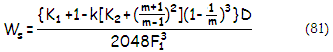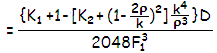With values for K1 and K2 satisfying Eq. 80, the result is zero value for Ws. In Eq. 81, the (K1+1) factor inside the main brackets is the aberration contribution of the primary, while the complex right-hand factor is the aberration contribution of the secondary. Deviations from values needed for a zero-sum in the four contributing elements shown in bottom relation - mirror conics, secondary location and radius of curvature - can either add up or partly offset one another. For instance, it is evident that change in the minimum relative secondary size k (i.e. change in its separation from the primary) will be mainly offset by a similar relative change in ρ (i.e. the secondary radius of curvature). But that will change secondary magnification.

Off-axis aberrations in two-mirror systems are coma, astigmatism and field curvature (distortion is usually negligible). General two-mirror system aberration coefficient for lower-order coma is a sum of the aberration contributions of the primary (Eq. 15.1) and secondary mirror (Eq. 15.2). After substitutions for the secondary's conic, stop (i.e. the primary) separation σ (FIG. 76, bottom) and the object (i.e. primary's image) distance factor Ω, in terms of the selected dimensionless parameters it can be written as: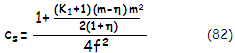Similarly, the two-mirror system aberration coefficient for lower-order astigmatism is: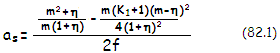with f being the system focal length. The P-V wavefront error at the best focus is, from Eq. 12 and Eq. 18, given by Wc=csαD3/12 and Wa=as(αD)2/4, respectively, with α being the field angle in radians.

Good approximation for the level of coma in a two-mirror system from Eq. 82 gives system coma approximately changing in proportion to [2+(K1+1)m3/(1+η)]/2F12, F1 being the primary mirror focal number F=ƒ1/D. For K1=-1 (paraboloidal primary), the coma changes as 1/F12, inversely to the square of the primary mirror F-number. For an aplanatic (coma-free) two-mirror system, needed primary mirror conic is approximated by K1~-1-2(1+η)/m3 (of course, coma of the primary is conic-independent as long as the stop is at its surface; the indication is indirect, due to primary's conic actually compensating for spherical aberration induced by aspherizing the secondary as needed to offset primary's coma).

Two-mirror system Petzval and best (median) image field curvature are: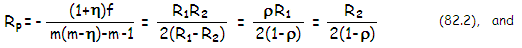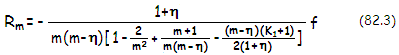respectively, with the system focal length ƒ being, as mentioned in the beginning, numerically positive for the Cassegrain and negative for Gregorian. For K1~-1, good approximation of the median field curvature, for η set to zero in the denominator, is given by: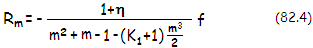The sign of Rm is negative for the Cassegrain, and positive for the Gregorian, indicating that median field curvature is concave toward secondary for the former, and convex for the latter.

Graph below shows Petzval and median field curvature for classical Cassegrain and Gregorian (K1=-1), for back focal length η=0.25 (note that the system focal length is positive for the Cassegrain and negative for the Gregorian, thus the sign of field curvature is as appears for the former, but opposite to it for the latter).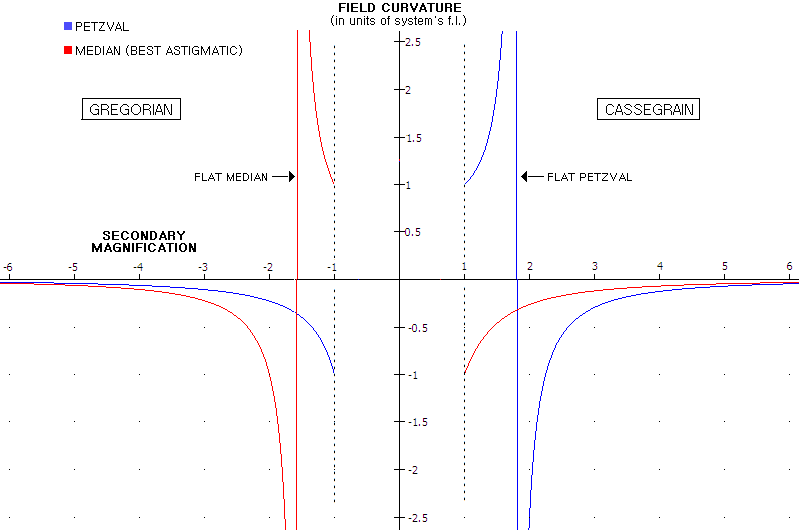Classical Cassegrain can have a flat Petzval surface, for m~1.8, but can't have a flat best astigmatic surface. When the Petzval is flat, the actual astigmatic best surface has curvature radius of 0.3
ƒ, ƒ being the system focal length. For m=1 (flat secondary) Petzval and median curvature are those of the primary, hence of the same magnitude but opposite in sign, equal in their absolute value to the system focal length.

On the other hand, Gregorian can't have flat Petzval (obviously, since employing two concave mirrors), but can have flat best astigmatic surface, for m~-1.6. However, such system is not practical, since the corresponding secondary is, from k=(1+η)/(m+1)=-2.083, more than twice the diameter of the primary. Practical Gregorian systems have m<-3.5, i.e. secondary's minimum size not larger than about 50% of the primary. For the same criterion, the lower magnification limit for the Cassegrain is m~1.5. Thus, while for given system ƒ-ratio the Gregorian will have flatter best image surface (and larger secondary) - its median curvature is nearly identical to the Petzval of the Cassegrain, and its Petzval is fairly similar to the Cassegrain's median curvature - for given primary's ƒ-ratio it is the Cassegrain that can reach faster system ƒ-ratio and have a flatter best image surface not only in terms of the system focal length, but in absolute terms as well. For instance, with an ƒ/3 primary the fastest that the Gregorian can go with the minimum 50% linear obstruction is ~ƒ/11, vs. ~ƒ/4.7 for the Cassegrain; the former has the best image surface radius ~0.15ƒ, and the latter ~0.45ƒ, or nearly 30% weaker.

Variations in the back focal length from 0.25 are relatively small for practical systems (even in the Nasmyth arrangement and η~0 there is no significant change in the median field curvature), which means that these plots are representative of the two systems in general.

Incident ray angle and the final image

Incident ray angle at any off-axis point in the final image of a two-mirror telescope is significantly larger than the corresponding incident angle at the aperture (primary mirror). This is a consequence of the ray reflected from the secondary appearing as if coming from the center of the exit pupil - image of the primary - projected by the secondary. Larger image forming angles are not an aberration factor in the final image itself, but are an aberration factor for the eyepiece, or other optical element following the image. Given system focal ratio, eyepiece field aberrations in a two-mirror system are larger than in a Newtonian, or a refractor.

Secondary mirror in a two-mirror system forms the system exit pupil (i.e. virtual image of the primary mirror) in front of the primary, at a distance E=(km2)/(m+k-1) from the final image, in units of the primary's focal length, with k being the height of marginal ray at the secondary (i.e. minimum secondary size) in units of the aperture radius, and m the secondary magnification.

Since the chief ray appears as if coming from the center of the exit pupil, the incident angle at the off-axis image point is given by h/E, h being its linear height in the image plane. At the same time, incident angle at the aperture is defined by h/ƒ, with ƒ=-mƒ1 being the system focal length  and ƒ1 the primary f.l. Consequently, magnification factor of the incident angle at the final image is given by ƒ/E or, after substituting for ƒ and E, by (m+k-1)/mk.

Thus, for average values of k~0.25 and m~4, the effective incident ray angle at the final image is over 3 times larger than the actual incident angle at the primary. It is greater for the Gregorian, since both, secondary magnification m and relative size k are numerically negative.

8.2. Two-mirror telescopes   ▐    8.2.2/3. Classical and aplanatic